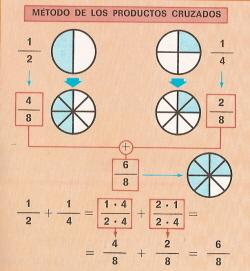Addition of fractions1.- Addition of fractions with the same denominator.

We have one piece of cake (1/5) and we add 2 pieces (2/5). Finally we have three pieces (3/5).

To add fractions with the same denominator, we must add the numerators, keeping the same denominator.

Examples: 2/7 + 3/7 + 1/7 = 6/7; 5/9 + 8/9 = 13/9

Solve these sums:

 2/7 + 3/7 = 3/5 + 1/5 = 1/4 + 3/4 = 3/8 + 1/8 + 2/8 =2.- Addition of fractions with different denominators.

First, we must reduce the two fractions to a common denominator: 1/2 + 1/4 = 4/8 + 2/8
Then, we sum 4/8 + 2/8 = 6/8

To add fractions with different denominators, we must reduce them to a common denominator and then, we must apply the previous rule.

Do these sums:

 1/2 + 1/3 = 1/3 + 1/4 = 1/2+2/3+3/4= 1/3 + 1/6 =

3.- Addition of mixed numbers

To add mixed numbers, we add the whole numbers and the fractional parts.

Examples 3 1/3+ 2 1/3 = (3 +2) and (1/3 + 1/3) = 5 2/3 1 1/4 + 2 1/3 = (1 +2) + (1/4 +1/3 = 3/12 + 4/12) = 3 7/12.

Do these sums of mixed numbers. Do it on paper and check it.

 3 y 3/5 + 2 y 1/3 = 2 y 1/3 + 1 y 1/5 = 7 y 1/4 + 6 y 1/3 = 3 y 1/9 + 2 y 1/7 =

4.- Simplified fractions.

If we divide the numerator and the denominator of a fraction by the same number, we obtain a simplified fraction.

Examples: 6/15 = 6:3 / 15:3 = 2/5; 8/12 = 4/6 = 2/3, 9/12 = 3/4.

Simplify these fractions:

 6/8 = 3/12 = 9/18 = 3/18 =

5- Add these fractions and simplify the result.

Do these operations on a paper.

Recuerda que 2/2 = 3/3 = 4/4 = 1

 1/4 + 3/4 = 3/8 + 1/8 = 1/2 + 1/4 = 1/6 + 1/6 =

6- Do these problems

Do it on a paper and check it:

 1. On Monday a worker won 8 1/3 euros and on Tuesday he won 9 2/5 euros. How much money did he win on both days? 2. John has 1/3 of a cake and Montse has 1/5. How many pieces of cake did they have in total? 3. Ana has 5 2/5 euros and Arturo has 6 1/4 euros. How much money do they have in total? 4. A boy drinks Į sip of a bottle and then he drinks 1/3. How much did he drink in total?

 If you are registered, please enter your data   Name:  Password:   | Educational applications | Mathematics | In Spanish  | To print
«Arturo Ramo GarcĒa.-Record of intellectual property of Teruel (Spain) No 141, of 29-IX-1999
Plaza Playa de Aro, 3, 1║ DO 44002-TERUEL